# Exploring Karplus-Strong Filters¶

#### Plucked String Synthesis¶

An example inspired by a talk given by Jim Rulla.

#### Imports¶

In :
# We needs some imports and also enable our inline plots
import matplotlib.pyplot as plt
import random
import wave
import StringIO
from IPython.core.display import HTML
import numpy

%matplotlib inline


#### Settings¶

In :
# Change these parameters to alter the sound

# Amplitude should be between 0.0 and 1.0
g_synth_amplitude = 1.0

# Desired frequency in Hz
g_synth_frequency_hz = 440

# Desired wave file duration in seconds
g_wav_duration_s = 4

# Specify the sample rate of the output wave file
g_wav_sample_rate = 44100

# Specify file name for wave file
#g_wav_filename = "karplus-strong-synth-%d.wav" % int(g_synth_frequency_hz)
g_wav_filename = "karplus-strong-synth.wav"

# Default fig sizes and dpi settings
g_figsize = (4,4)
g_dpi = 100

#***
# Calculated globals
# You shouldn't need to alter these as they are based on the
# settings you choose above.
#***
g_synth_period_s = 1.0 / g_synth_frequency_hz
g_synth_period_samples = int(g_synth_period_s * g_wav_sample_rate + 0.5)
g_synth_duration_samples = int(g_wav_duration_s * g_wav_sample_rate)
print "Synth period: %f sec." % g_synth_period_s
print "\tor %d samples." % g_synth_period_samples
print "Wav duration: %f sec." % g_wav_duration_s
print "\tor %d samples." % g_synth_duration_samples

Synth period: 0.002273 sec.
or 100 samples.
Wav duration: 4.000000 sec.
or 176400 samples.


#### Functions¶

In :
# Generate a list of random samples
def gen_random_samples(amplitude, num_samples):
A = int(amplitude * 32767.0 + 0.5)
samples =  * num_samples
for n in range(0, len(samples)):
samples[n] = int(-A if random.random() > .5 else A)
return samples

In :
# String filter
def make_string_sound(impulse, duration_samples):
p = len(impulse)
output = []
output.extend(impulse)
output.extend([int(0)] * (duration_samples - p))

for i in range(p, len(output)):
output[i] = int(((output[i - p] + output[i - p + 1]) / 2.0) + 0.5)

return output

In :
# Drum filter
def make_drum_sound(impulse, duration_samples, blend_factor):
"""blend_factor should be between 1 and 0.
A blend_factor of 1 produces the same result as the
make_string_sound()"""
p = len(impulse)
output = []
output.extend(impulse)
output.extend([int(0)] * (duration_samples - p))

for i in range(p, len(output)):
factor = 2.0 if random.random() <= blend_factor else -2.0
output[i] = int(((output[i - p] + output[i - p + 1])/ factor) + 0.5)

return output

In :
# Generate data suitable to writing to wave file
def sound_to_bytes(sound):
"""Takes a list containing sound samples and converts to a buffer of bytes"""
output = StringIO.StringIO()
for sample in sound:
output.write(chr(sample & 0xFF))
output.write(chr((sample >> 8) & 0xFF))
return output.getvalue()


#### Generate an Impulse¶

In :
# Seed our random number generator
random.seed()

# Generate our impulse
impulse = gen_random_samples(g_synth_amplitude, g_synth_period_samples)

# Show our random impulse
plt.figure(figsize=g_figsize)
plt.plot(range(0, len(impulse)), impulse)
plt.title("Initial Impulse")
plt.grid()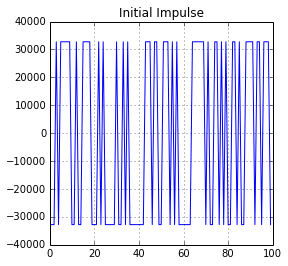#### Feed Through the Filter¶

In :
# Synthesize through filter
sound = make_string_sound(impulse, g_synth_duration_samples)
#sound = make_drum_sound(impulse, g_synth_duration_samples, 1)

# Show our sound
plt.figure(figsize=g_figsize)
plt.plot(range(0, len(sound)), sound)
plt.title("Synthsized signal @ %dHz" % g_synth_frequency_hz)
plt.grid()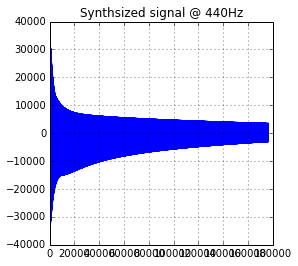In :
# Show a zoomed view
start = 20000 #2 * g_synth_period_samples
end = start + 400
plt.figure(figsize=g_figsize)
plt.plot(range(start, end), sound[start:end])
plt.title("Zoomed View")
plt.grid()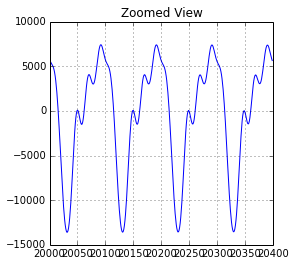#### FFT¶

In :
# Look at an FFT of only the synthesized portion
p = 2 * len(impulse)
fft = [abs(f) for f in numpy.fft.rfft(sound[p:p + g_wav_sample_rate], g_wav_sample_rate)]
fft = 0 # Ignore DC
freqs = [((g_wav_sample_rate / 2.0) / len(fft)) * n for n in range(0, len(fft))]

# Entire FFT
fig, axes = plt.subplots(figsize=g_figsize, dpi=g_dpi)
axes.plot(freqs, fft)
axes.set_title("FFT Magnitudes")
axes.set_xlabel("Hz")
axes.grid()

# First part of FFT
fig, axes = plt.subplots(figsize=g_figsize, dpi=g_dpi)
axes.plot(freqs[0:1000], fft[0:1000])
axes.set_title("FFT Magnitudes")
axes.set_xlabel("Hz")
axes.grid()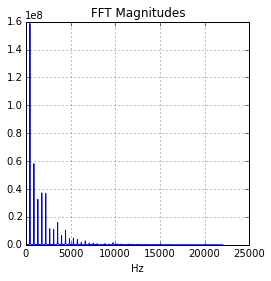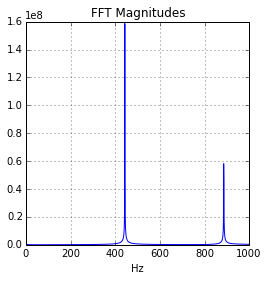#### Save and Play Sound¶

In :
# Wave files are easy to make, so let's do that!
wf = wave.open(g_wav_filename, "w")
wf.setnchannels(1)
wf.setsampwidth(2)
wf.setframerate(g_wav_sample_rate)
wf.writeframes(sound_to_bytes(sound))
wf.close()

# Generate some HTML to wrap up the audio file!
# Should even show up in exported HTML
wav_encoded = wav.encode("base64")
audio_tag = """
<audio controls="controls" style="width:600px" >
<source src="data:audio/wav;base64,{0}" type="audio/wav"/>
Your browser does not support the audio element.
</audio>
""".format(wav_encoded)
HTML(audio_tag)

Out:

# Resources¶